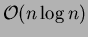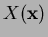Next: Applications in Computational Mechanics Up: A Note on Natural Previous: Implementation of the Natural

# A Study in Contrast: FE, EFG, and NEM

A comparison of FEM, EFG, and NEM follows:
1. The interpolant in both FEM and NEM possess similar properties (see Section 3), and the interpolants are exactly the same in 1D. The EFG trial function does possess linear consistency but is not an interpolant; hence, Lagrange multipliers or a coupling with FE is required to satisfy the essential boundary conditions.
2. A similar'' (in principle) mesh structure (triangulation) is used in both FE and NEM, while the approximant in EFG (a meshless method'') is constructed solely on the basis of a set of nodes and a weight function with compact support.
3. In all three methods, the interpolant/approximant has a local character because of the compact support of the shape functions.
4. In FE, the functional that is integrated over the domainin the weak form is constructed using a polynomial trial function and hence we can obtain an accurate estimate of the integral using Gauss-Legendre quadrature (within machine precision and round-off errors). As opposed to FE, in both NEM and EFG (moreso probably in EFG), the shape functions are not polynomials in general. Hence numerical integration is an issue and the question of optimum/accurate quadrature arises, which is still an open issue. In , three-point Gauss-Legendre quadrature was found to be sufficient to obtain accurate results.
5. In the discrete system, the stiffness matrixis banded in FEM, while in both EFG and NEM,is not banded in general.
6. The computational cost (apart from numerical issues) is an important factor in the feasibility and usability of a numerical method. Finite elements, apart from their nice local (polynomial) properties, are a computationally attractive choice because of the very fast execution times that are attainable. The key time-consuming steps in the NEM are:
1. Dirichlet tessellation (Voronoi diagram) of the nodes -- for some typical algorithms, see [17,18,19]. In 2D, Fortune's sweepline' algorithm  is considered to be one of the fastest, while in higher dimensions, the Qhull package  based on the quickhull' algorithm  appears to be the unanimous choice. Both the above arealgorithms.
2. Search for natural neighbors for a sampling point. The walking-triangle algorithm due to Lawson , which is aalgorithm, is a suitable choice.
 used the Qhull package  for the Dirichlet tessellation and Lawson's algorithm  for the neighbor-search. They have reported  execution times for NEM that are about a factor of two slower than quadratic finite elements.Next: Applications in Computational Mechanics Up: A Note on Natural Previous: Implementation of the Natural
N. Sukumar